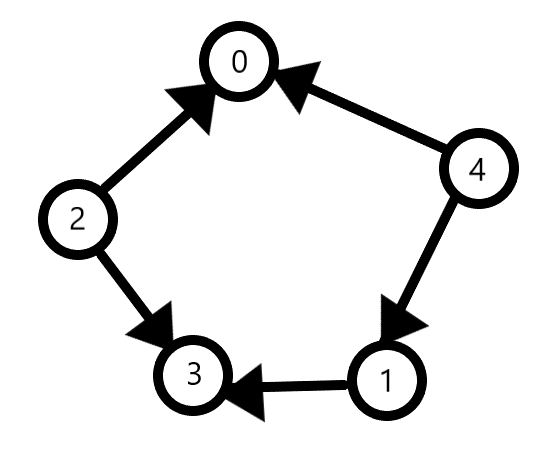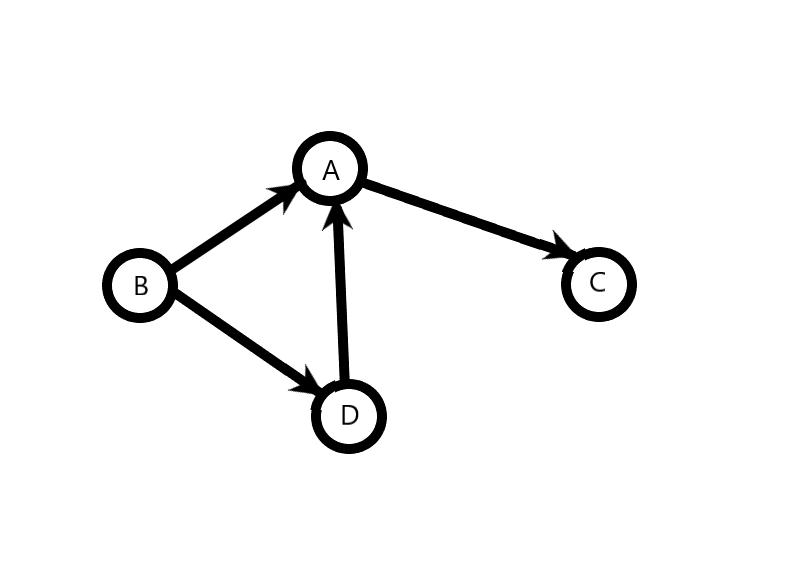×

Search anything:

# Alien Dictionary problem: Sorted order of characters

#### Algorithms Graph AlgorithmsIn this article, we are given a sorted list of words in some alien dictionary. Based on the list, we have to generate the sorted order of characters in that alphabet. This problem can be solved using the idea of Topological Sort and Direct Acyclic Graphs. So, let's begin!

1. Understanding Topological Sort
2. Alien Dictionary Problem
3. What we are supposed to do
4. Our approach
5. Time and Space Complexity

Prerequisite: Topological Sort

This is similar to Leetcode problem 269. Alien Dictionary.

## Understanding Topological Sort

Topological Sort in a directed acyclic graph is a sorted order of vertices in which if u--->v is a directed edge in the graph from u to v, then u comes before v in the sort.

Suppose we have a graph:In this case, Vertices 2 and 4 come earliest as they have no incoming edges. 1 comes before 3 as we have an edge from 1 to 3. 3 and 0 come at the end since they have no outgoing edges. So, we arrive at the order: 4 2 1 3 0.
The same could easily be written as 2 4 1 0 3. The order of 2 and 4 makes no difference as there are no edges between them.

A graph can have multiple possible topological sorts. The algorithm for finding the topological sort uses the depth first traversal and a queue data structure as well as an indegree array, which stores the number of edges which are directed inward to a particular vertex.

Let us quickly see the C++ implementation for this:

``````	vector<int> topoSort(int V, vector<int> adj[])
{

vector<int> ans; queue<int> q; vector<int> indegree(V,0);
for(int i=0;i<V;i++){
indegree[x]++;
}
}
for(int i=0; i<V;i++){
if(indegree[i]==0){
q.push(i);
}
}
while(!q.empty()){
int n=q.front();
q.pop();
ans.push_back(n);
indegree[x]--;
if(indegree[x]==0){
q.push(x);
}
}
}
return ans;
}
``````

## Alien Dictionary Problem

Problem Statement: Given a sorted alien language dictionary with N words and k initial alphabets from a standard English dictionary, determine the alien language's character order.

So, we are given a vector strings in some alien language (which also uses the letters of the english alphabet) which are sorted in the order in which they'd appear in the dictionary of that language. Now, our motive is to examine these given strings and find the order in which the letters occur in that alien language. For example, the order of letters in english goes A B C D... Z. The order in the alien language can be something else entirely, like B A C H...F.

The order of letters will be: B D A C.

Dictionary Order={"caa","aaa","aab"}.
The order of letters will be: C A B.

Dictionary Order={"aa","aaa"}.
The order of letters will be: A.

Dictionary Order={"ab","aaa"}.
The order of letters will be: B A.

## What we are supposed to do

In the first test-case, compare the first two strings. They vary at the first letter. So, we infer that B comes before A in the dictionary. The letters after this do not contribute to our observations. Compare strings two and three. "abc" is common in both terms. They vary at "d" and "a", implying that D comes before A. Similarly, A comes before C and B comes before D.
From these, we arrive at the order B D A C.

Repeat these observations for all the other test-cases to gain a proper understanding of what we are aiming to achieve.
In case of test-case 3, we have "aa" and "aaa". In dictionary order, the shorter word "aa" comes before "aaa".

## Our approach

On comparison of strings, we find various dependencies between characters. We shall represent these dependencies using a Directed Graph where an edge goes from the character which appears before to the character which appears after. So, for the first test-case, we have the following graph.After this, we are going to apply the topological sort on the graph to find the order of characters in the dictionary. We get B D A C, which is the required solution.

So, we have:

1. Iterate through the given vector of strings and compare every consecutive pair of strings u and v letter by letter. The moment the letters mismatch, we add this edge to the graph, from the letter in u to the letter in v.
2. The directed acyclic graph is ready now. Apply topological sorting to it and find the order of characters.

Let us look through the C++ implementation of this. First up, we have the function `find_order()`, which takes in two parameters, the array of strings `given[]` and the number of strings `n`. It traverses the array, compares consecutive strings and when a character mismatch is found, adds the edge to the graph g, which we are implementing using an unordered map.

`````` string findOrder(string given[], int n) {
int i = 0, j = 0;
unordered_map < char, vector < char >> g;
for (i = 0; i < n - 1; i++) {
int m = min(given[i].size(), given[i + 1].size());
for (j = 0; j < m; j++) {
if (given[i][j] != given[i + 1][j]) {
g[given[i][j]].push_back(given[i + 1][j]);
break;
}
}
}
}
``````

In the return statement we have called the function `toposort()`, which we shall define now. `toposort()` takes in two parameters, an adjacency list `g` and the string array `given[]`.

It uses a map to store the indegree of the characters in the graph and a queue data structure to implement topological sort. It returns a string `ans` which is the solution.

``````string toposort(unordered_map < char, vector < char > > g, string given[]) {
queue < char > q;
unordered_map < char, int > indegree;
string ans = "";
for (auto i: g) {
for (char x: g[i.first]) {
indegree[x]++;
indegree[i.first] += 0;
}
}
for (auto i: indegree) {
if (i.second == 0) {
q.push(i.first);
}
}
while (!q.empty()) {
char n = q.front();
q.pop();
ans.push_back(n);
for (int x: g[n]) {
indegree[x]--;
if (indegree[x] == 0) {
q.push(x);
}
}
}
// For unary languages
if (ans == "") {
ans.push_back(given);
}
return ans;
}
``````

The function first calculates the indegree of every character. Then, all vertices with indegree 0 are pushed into the queue. Then, topological sort is begun, the adjacency list is traversed and indegree of all visited vertices is decreased by 1 with each visit. If indegree becomes 0, it's inserted in the queue. Every letter popped from the queue is appended to the string ans which is initiated with "".

Notice the code segment titled "For Unary Languages". Unary languages are those which have only one letter in the aplhabet. Example, the test-case "aa","aaa","aaaa". Here, since only one character is used, we cannot have a possible directed acyclic graph. This implies that our topological sort shall fail and we will be returned "" as the answer.

But we know that the alphabet consists of the letter 'a'. To account for this, we add the given `if` condition.

Let's take a look at our overall code:

``````#include <bits/stdc++.h>
using namespace std;

string toposort(unordered_map < char, vector < char > > g, string given[]) {
queue < char > q;
unordered_map < char, int > indegree;
string ans = "";
for (auto i: g) {
for (char x: g[i.first]) {
indegree[x]++;
indegree[i.first] += 0;
}
}
for (auto i: indegree) {
if (i.second == 0) {
q.push(i.first);

}
}
while (!q.empty()) {
char n = q.front();
q.pop();
ans.push_back(n);
for (int x: g[n]) {
indegree[x]--;
if (indegree[x] == 0) {
q.push(x);
}
}
}
if (ans == "") {
ans.push_back(given);
}
return ans;
}
string findOrder(string given[], int n) {
int i = 0, j = 0;
unordered_map < char, vector < char > > g;
for (i = 0; i < n - 1; i++) {
int m = min(given[i].size(), given[i + 1].size());
for (j = 0; j < m; j++) {
if (given[i][j] != given[i + 1][j]) {
g[given[i][j]].push_back(given[i + 1][j]);
break;
}
}
}
}

int main() {
string given[] = {
"baa",
"abcd",
"abca",
"cab",
};
int n = 5;
cout << findOrder(given, n);
return 0;
}
``````

The output received is bdac which is the correct solution.

# Time and Space Complexity

## Time complexity

In our approach, let n be the number of words given and m be the no. of characters in each word. Then, when we compare consecutive words for mismatches, every word is compared to this predecessor and successor, character by character. So, the time complexity for this comparison will be of the order of total number of characters in the words i.e. O(m * n).

This is followed by the topological sort. Let there be d characters in the alphabet. Then, the number of edges will be n-1 at most. So, time complexity for this topological sort will be O(vertices + edges) i.e. O(d+n-1) => O(d+n).
Overall complexity of our approach will be: O(m * n) + O(d+n)= O(m * n).

## Space Complexity

Firstly, when we create the graph using adjacency list, the time complexity will be O(vertices + edges). Here, vertices will be d, the number of characters in the alphabet and edges will be n-1 at most, where n is the number of words given. So, space complexity is O(d+n-1) = O(d+n).

This is followed by topological sort, where we use a map indegree, which will take O(d) space and a queue, which will also take around O(d) in the worst case. So, space complexity for sorting is O(d) + O(d)= O(d).

Overall space complexity for this approach will be O(d+n) + O(d) = O(d+n).

Thus, in this article at OpenGenus, we have solved the alien dictionary problem using concepts of directed acyclic graphs and topological sorting. Keep learning!#### J. Varun Iyer

J. Varun Iyer is a Student at NIT Raipur and an Intern at OpenGenus.

Alien Dictionary problem: Sorted order of characters